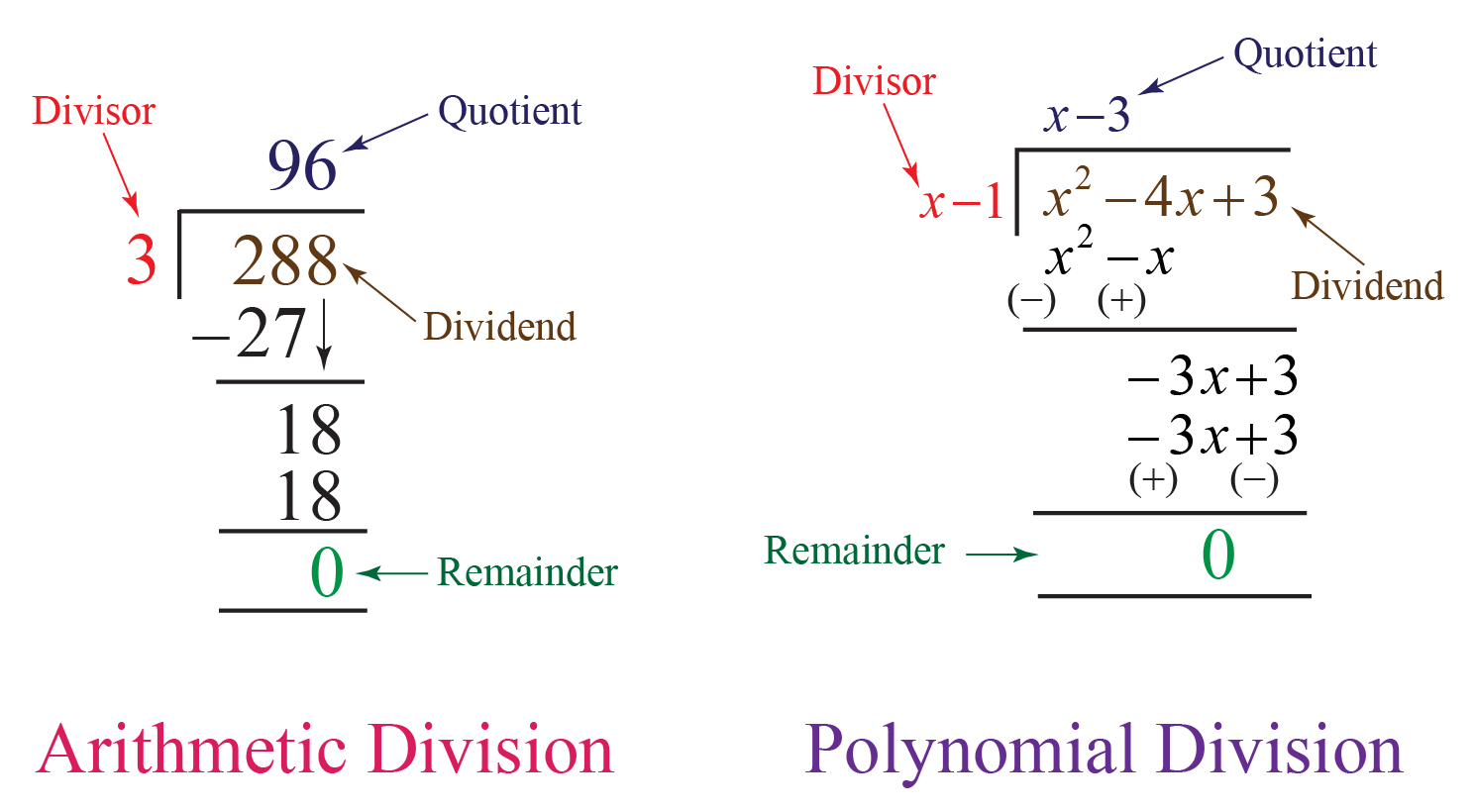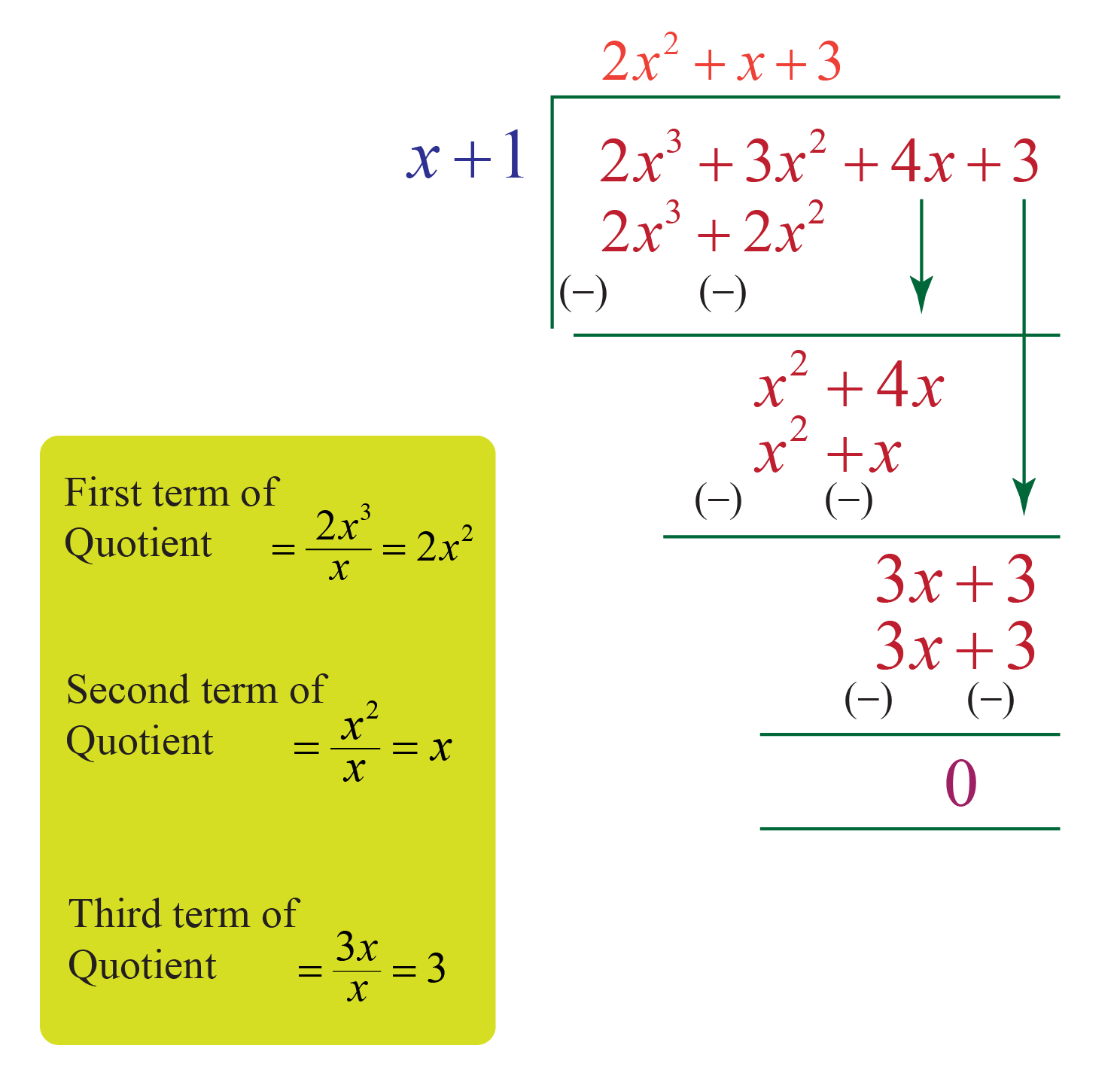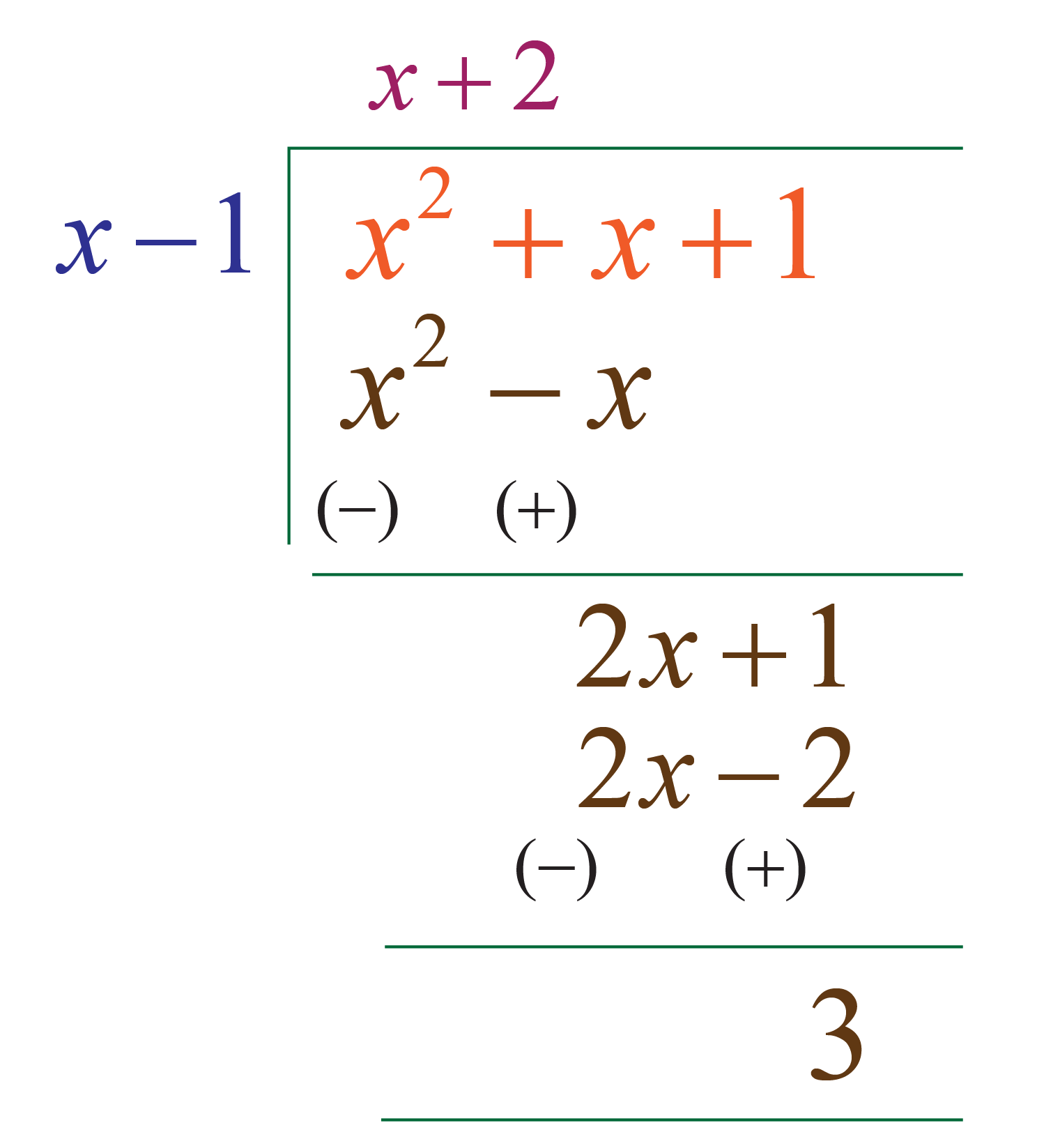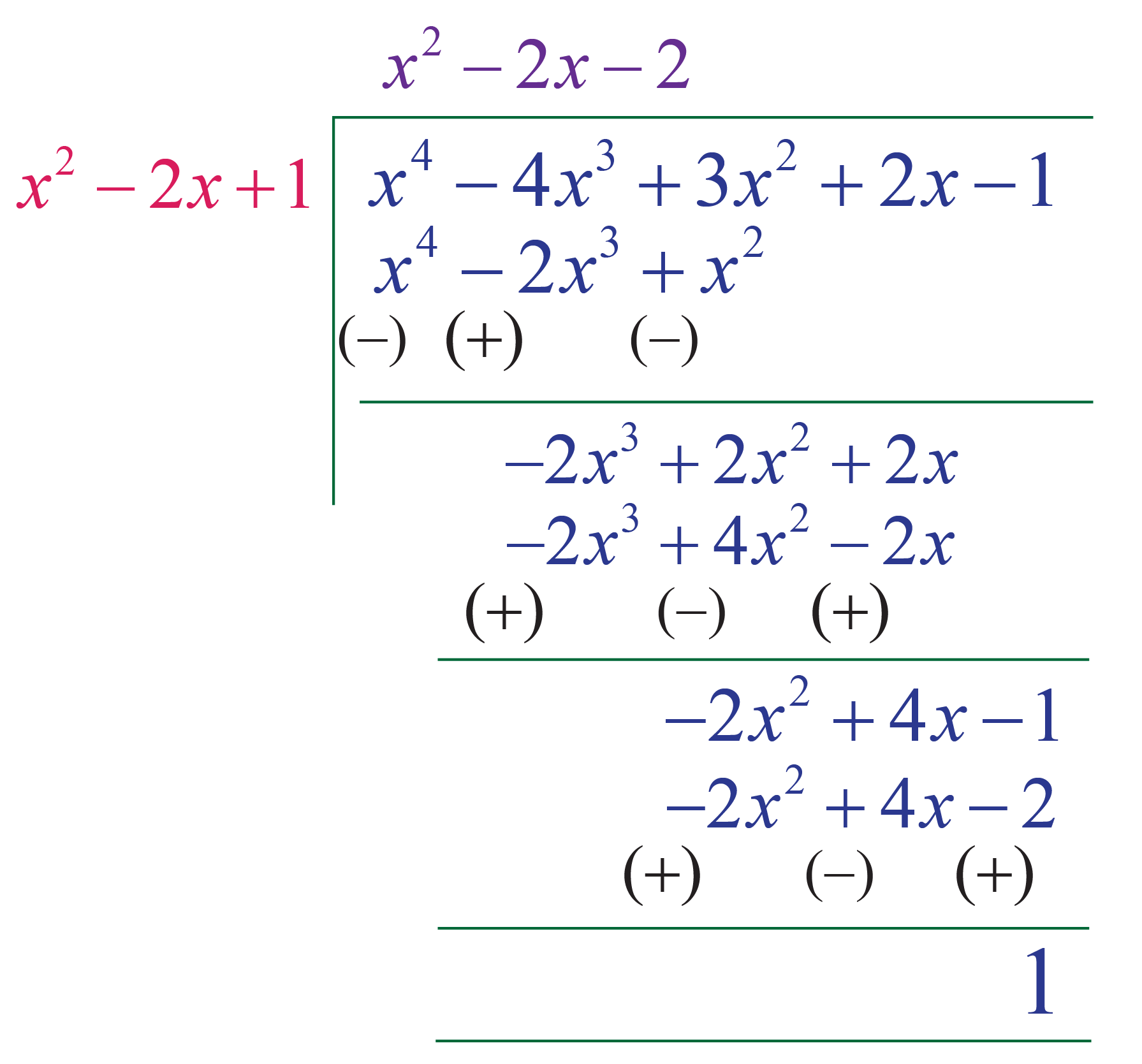# Division Algorithm for Polynomials

Division Algorithm for Polynomials

Division is an arithmetic operation that involves grouping objects into equal parts. It is also understood as the inverse operation of multiplication.

For example, in multiplication, 3 groups of 6 make 18

Now, if 18 is divided into 3 groups, it gives 6 objects in each group.

Here 18 is the dividend, 3 is the divisor and 6 is the quotient.

Dividend is the product of the divisor and the quotient, added to the remainder (if any). The same rules apply for the division of polynomials as well.

The division of polynomials involves dividing one polynomial by a monomial, binomial, trinomial or a polynomial of a lower degree. In a polynomial division, the degree of the dividend is greater than the divisor. To verify the result, we multiply the divisor and the quotient and add it to the remainder, if any.## Lesson Plan

 1 What Is Division Algorithm of Polynomials? 2 Important Notes on Division Algorithm of Polynomials 3 Solved Examples on Division Algorithm of Polynomials 4 Tips and Tricks on Division Algorithm of Polynomials 5 Interactive Questions on Division Algorithm of Polynomials

## What Is Division Algorithm of Polynomials?

The Division algorithm for polynomials says, if p(x) and g(x) are the two polynomials, where g(x)≠0, we can write the division of polynomials as:

 \begin{align}p(x) = q(x) \times g(x) + r(x)\end{align}

where, \begin{align}p(x)\end{align} is the dividend.

\begin{align}q(x)\end{align} is the quotient.

\begin{align}g(x)\end{align} is the divisor.

\begin{align}r(x)\end{align} is the remainder.

If we compare this to the regular division of numbers, we can easily understand this as: Dividend = (Divisor X Quotient) + Remainder

## Procedure To Divide a Polynomial by Another Polynomial

The steps for the polynomial division algorithm are given below.

Step 1: Arrange the dividend and the divisor in the descending order of their degrees.

Step 2: Find the first term of the quotient by dividing the highest degree term of the dividend with the highest degree term of the divisor.

Step 3: Find the second term of the quotient by dividing the largest degree term of the new dividend obtained in step 2 with the largest degree term of the divisor.

Step 4: Repeat the steps again until the degree of the remainder is less than the degree of the divisor.

Let us understand this with an example.

Divide \begin{align}2x^3+3x^2+4x+3\end{align} by  \begin{align}x+1\end{align}

Here

\begin{align}p(x) = 2x^3+3x^2+4x+3\end{align}

\begin{align}g(x) = x+1\end{align}

Let us follow the division algorithm steps to perform the polynomial division.

Step 1 : The polynomials are already arranged in the descending order of their degrees.

Step 2: The first term of the quotient is obtained by dividing the largest degree term of the dividend with the largest degree term of the divisor.

\begin{align}\therefore First \: term = \dfrac{2x^3}{x}\end{align}  \begin{align} = 2x^2\end{align}

Step 3: The new dividend is \begin{align}x^2+4x\end{align}

Step 4: The second term of the quotient is obtained by dividing the largest degree term of the new dividend obtained in step 2 with the largest degree term of the divisor.

\begin{align}\therefore Second \: term = \dfrac{x^2}{x}\end{align}  \begin{align} = x\end{align}

Step 5: The new dividend is  \begin{align}3x+3\end{align}

Step 6: The third term of the quotient is obtained by dividing the largest degree term of the new dividend obtained in step 4 with the largest degree term of the divisor.

\begin{align}\therefore Third \: term = \dfrac{3x}{x}\end{align}  \begin{align} =3\end{align}

Step 7: The quotient is \begin{align}2x^2+x+3\end{align}\begin{align}p(x) = 2x^3+3x^2+4x+3\end{align} is the dividend

\begin{align}q(x) = 2x^2+x+3\end{align} is the quotient

\begin{align}g(x) = x+1\end{align} is the divisor

\begin{align}r(x) = 0 \end{align} is the remainder

### Divison Algorithm For Linear Divisors

When a polynomial of degree  \begin{align}n \geq 1 \end{align}  is divided by a divisor with degree 1, then we call it as a division by linear divisor.  The division algorithm for linear divisors is the same as that of the polynomial division algorithm discussed above except the fact that the divisor is of the degree 1.

Let us look at an example below.

Let

\begin{align}p(x) = x^2+ x + 1 \end{align} be the dividend

and  \begin{align}g(x) = x-1\end{align} be the divisor

Here the degree of the divisor is 1. Here  \begin{align}g(x) = x-1\end{align} is called a "Linear divisor".Divison Algorithm For General Divisors

Division algorithm for general divisors is the same as that of the polynomial division alogorithm discussed under the section of division of one polynomial by another polynomial. One important fact about this division is that the degree of the divisor can be any positive integer lesser than the dividend.

Let us take an example.

Let

\begin{align}p(x) = x^4- 4x^3 + 3x^2+ 2x - 1 \end{align} be the dividend

and  \begin{align}g(x) = x^2- 2x + 1\end{align} be the divisor

Here the degree of the divisor is 2, which is lesser than the dividend's degree.More Important Topics
Numbers
Algebra
Geometry
Measurement
Money
Data
Trigonometry
Calculus
More Important Topics
Numbers
Algebra
Geometry
Measurement
Money
Data
Trigonometry
Calculus